## Plus Blog

December 12, 2013

There's nothing simpler than 1,2,3, ... we understand these numbers instinctively and that's why they're called the natural numbers. But if you really think about it, what are these numbers? How would you describe them to an alien devoid of a number instinct? Here's one way of defining them, developed by the Italian mathematician Giuseppe Peano: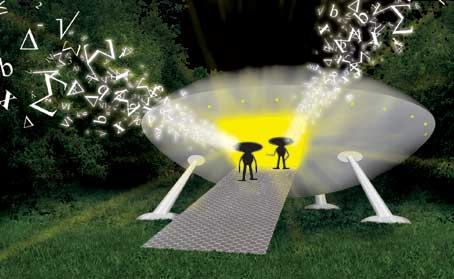Explaining numbers to aliens.

1. First you proclaim that 1 is a natural number
2. Then you say that every natural number n has a successor s(n), which you can also write as n+1.
3. We also insist that this successor is never equal to 1
4. And that different numbers have different successors.

These four rules give you all the natural numbers, neatly ordered in a line, starting from 1 (you could also have started from 0). They also give you arithmetic, since addition and multiplication are about repeatedly adding 1s and you know how to do this: you simply move up to the successor of the number you're looking at. Subtraction and division are just the reverse of addition and multiplication. So equipped with these rules your innumerate alien could actually do some pretty decent number theory.

The four rules form the basis of what's called Peano arithmetic. It's a formal mathematical system based on a set of axioms (which includes these four rules) together with a language in which to speak about numbers and rules for logical inference. In the beginning of the 20th century mathematicians hoped they could turn all of maths into one giant formal system similar to Peano's arithmetic. That way they could prove everything directly from the axioms, without any hidden assumptions, and make sure that maths contains no contradictions. But their dream was shattered in the 1930s by the logician Kurt Gödel, who showed that there are logical limits to what you can do using formal systems. Find out more in

December 10, 2013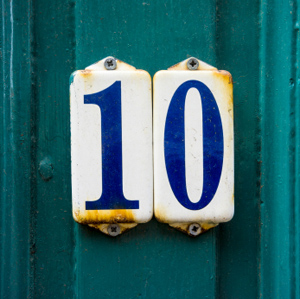If it wasn't for the Babylonians who lived millennia ago, paying your bill at a restaurant or checking your credit card bill would be much more painful than it already is. And so would anything else involving numbers. It was these Babylonian scholars who had the brilliant idea of making the value of a numeral dependent on its position in a string of numerals: if you see a 1 at the end of a string, you know it means 1, if it's shifted one to the left, you know it means 10, if it's shifted two to the left, it means 100 and so on. This means that using only the symbols 0 to 9 you can write down every single whole number, no matter how large, rather than having to invent a new symbol every time you go up a order of magnitude.

The Babylonian number system actually had 60 as its base, rather than base ten as we do. The numerals we use today, including the use of a symbol (zero) for nothing instead of a gap, stems from India.

Find out more on in the Fabulous positional system and out other articles about number systems.

December 9, 2013

I went Christmas shopping yesterday, to buy a book from the local bookshop that costs £7. I didn't have any money, so I borrowed £5 from Marianne and £5 from Owen. I bought the book and got £3 change. I gave £1 back to each of my kind colleagues and I keep the remaining £1. I now owe each of them £4 and I have £1, giving £9 in total. But I borrowed £10. Where's the missing pound?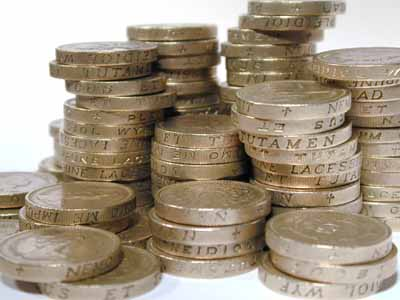There it is!

The answer is that the £10 are a red herring. There's no reason why the money I owe after the whole transaction and the money I still have should add up to £10. Rather, the money I owe minus the change I got should come to the price of the book, that is £7. Giving a pound back to each of Marianne and Owen just re-distributes the amounts. The money I still owe is reduced to £8 and the money I still have to £1. Rather than having £10-£3=£7, we now have £8-£1=£7. Mystery solved!

December 8, 2013

An infinite set is called countable if you can count it. In other words, it's called countable if you can put its members into one-to-one correspondence with the natural numbers 1, 2, 3, ... . For example, a bag with infinitely many apples would be a countable infinity because (given an infinite amount of time) you can label the apples 1, 2, 3, ... and so on.

Two countably infinite sets A and B are considered to have the same "size" (or cardinality) because you can pair each element in A with one and only one element in B so that no elements in either set are left over. This idea seems to make sense, but it has some funny consequences. For example, the even numbers are a countable infinity because you can link the number 2 to the number 1, the number 4 to 2, the number 6 to 3 and so on. So if you consider the totality of even numbers (not just a finite collection) then there are just as many of them as natural numbers, even though intuitively you'd think there should only be half as many.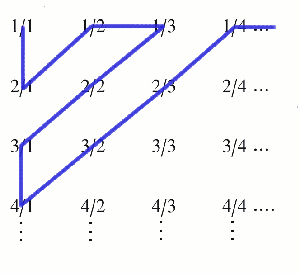Something similar goes for the rational numbers (all the numbers you can write as fractions). You can list them as follows: first write down all the fractions whose denominator and numerator add up to 2, then list all the ones where the sum comes to 3, then 4, etc. This is an unfailing recipe to list all the rationals, and once they are listed you can label them by the natural numbers 1, 2, 3, ... . So there are just as many rationals as natural numbers, which again seems a bit odd because you'd think that there should be a lot more of them.

It was Galileo who first noticed these funny results and they put him off thinking about infinity. Later on the mathematician Georg Cantor revisited the idea. In fact, Cantor came up with a whole hierarchy of infinities, one "bigger" than the other, of which the countable infinity is the smallest. His ideas were controversial at first, but have now become an accepted part of pure mathematics.

You can find out more about all this in our collection of articles on infinity.

December 7, 2013We've all got favourite numbers and some of us even go as far as thinking some numbers lucky or unlucky. Seven is probably one that crops up quite a lot in this context, so it's nice to know that it really is a lucky number. No, seriously, that's true! There is a mathematical recipe for finding lucky numbers, and 7 is one of them.

The recipe goes like this. Write down all the positive whole numbers starting from 1. First, you eliminate all even numbers, leaving only the odds:

1, 3, 5, 7, 9, 11, 13, 15, ... .

The next number on the list after 1 is 3, so you now go and eliminate every third number, leaving:

1, 3, 7, 9, 13, 15, ... .

The next surviving number after 3 is 7, so you eliminate every 7th number in the list of survivors. And so on. When you've finished this process the sequence of surviving numbers starts:

1, 3, 7, 9, 13, 15, 21, 25, 31, 33, 37, 43, 49, 51, 63, 67, 69, 73, 75, 79, 87, 93, 99, ... .

These surviving numbers are called lucky numbers. They are similar to the prime numbers in some ways. There are infinitely many of them, and they are the result of a sieving process (successively eliminating numbers) similar to the sieve of Eratosthenes which sieves out primes. When you try and approximate the distribution of the lucky numbers among the other numbers as you move up the number line, the result is akin to the prime number theorem. The lucky numbers even have their own version of the Goldbach conjecture, which states that any odd number greater than 4 can be written as the sum of two primes. One thing that isn't known, though, if whether there are infinitely lucky numbers that are also prime, or only finitely many.

All this doesn't make 7 lucky in the everyday sense of the word — but it makes us feel very lucky to have so many numbers to play with!

December 6, 2013

Now here's an important mathematical question: how likely are you to win the lottery?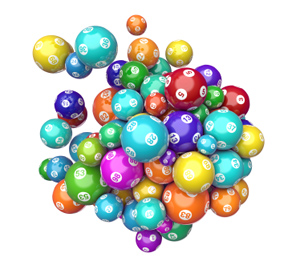1 in 14 million.

In the UK lottery you have to choose 6 numbers out of 49, and for a chance at the jackpot you need all of your 6 numbers to come up in the main draw. So the question is really how many possible combinations of 6 numbers can be drawn out of 49? There are 49 possibilities for the first number, 48 for the second, and so on, to 44 possibilities for the sixth number, so there are 49x48x47x46x45x44=10,068,347,520 ways of choosing those six numbers... in that order.

But we don't care which order our numbers are picked, and the number of different ways of ordering 6 numbers are 6x5x4x3x2x1=6! =720: there are six possibilities for the number that comes first in the list, 5 for the number that comes second, and so on. Therefore our six numbers are one of 49x48x47x46x45x44/6! = 13,983,816 so we have about a one in 14 million chance of hitting the jackpot. Hmmm...

But on a brighter note, we have just discovered a very useful mathematical fact: the number of combinations of size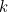(sets of objects in which order doesn’t matter) from a larger set of size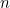is: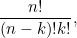where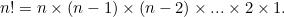This sort of argument lies at the heart of combinatorics, the mathematics of counting. It might not help you win the lottery, but it might keep you healthy. It is used to understand how viruses such as influenza reproduce and mutate, by assessing the chances of creating viable viruses from random recombination of genetic segments.

You can read more on the uses of combinatorics, including in money matters (lottery), love (well, kissing frogs) and fun (juggling and Rubik's cubes):

Combinatorics on Plus